# 3. Use Laplace transforms to solve the following initial value problems. Write the solution (t) f...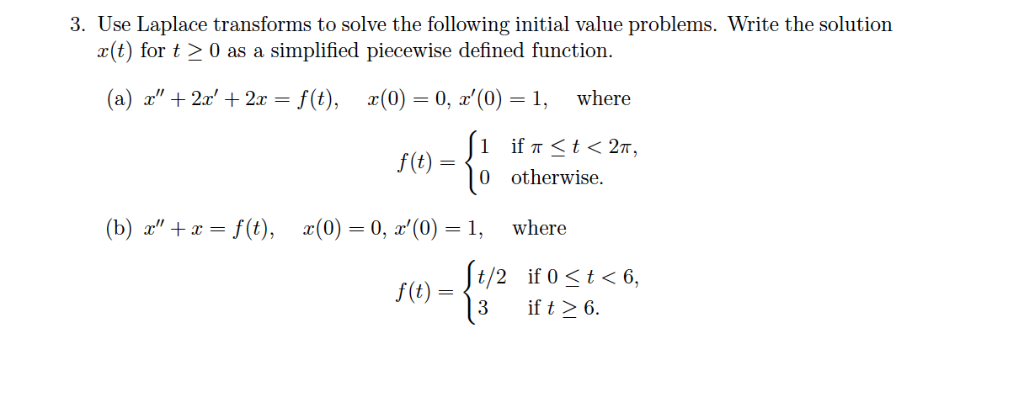3. Use Laplace transforms to solve the following initial value problems. Write the solution (t) for t20 as a simplified piecewise defined function. (a) z', + 2x' + 2x-f(t), x(0-0, z'(0)-i, where f(t)-〈0 otherwise. (b) z', +x-f(t), x(0) 0, z'(0)=1, where t/2 if 0 t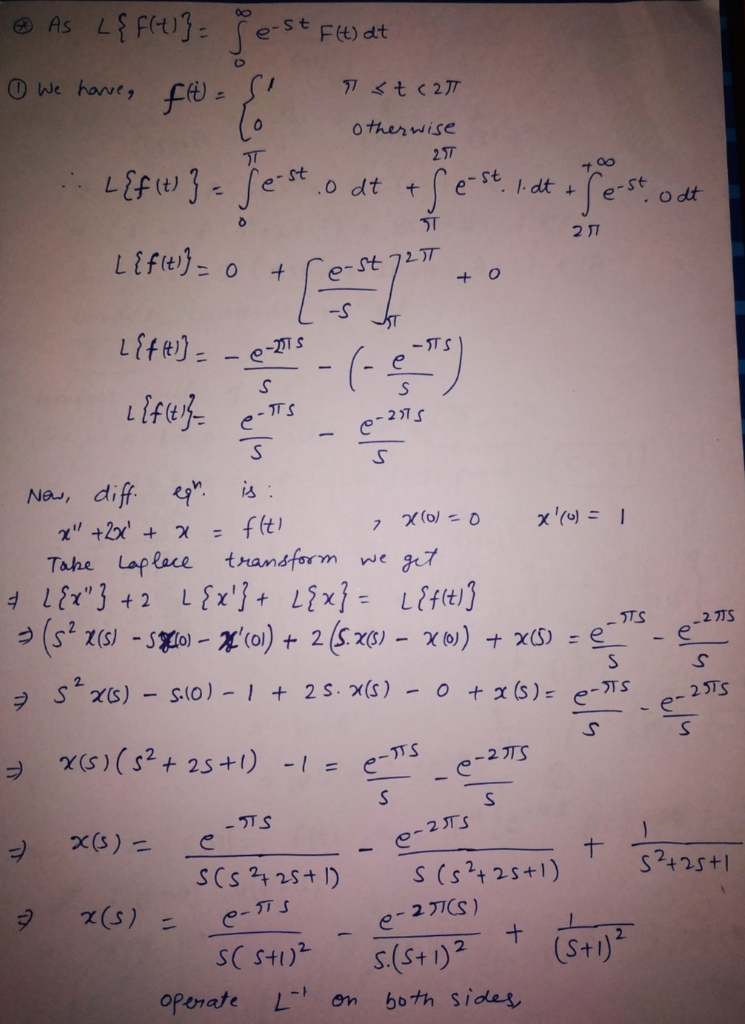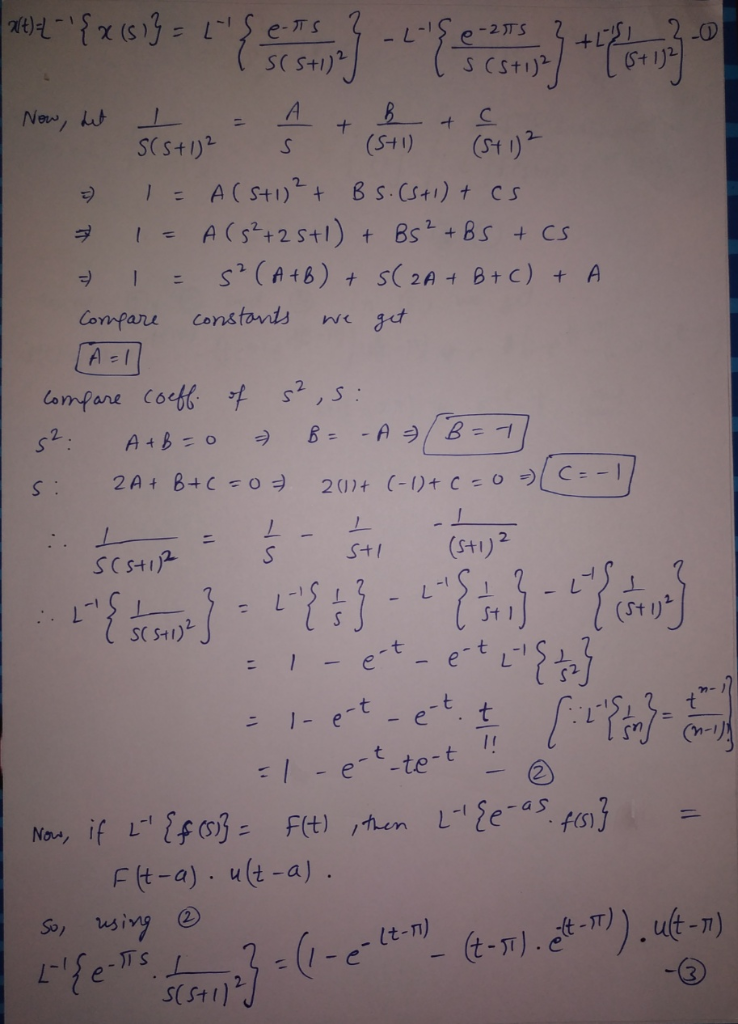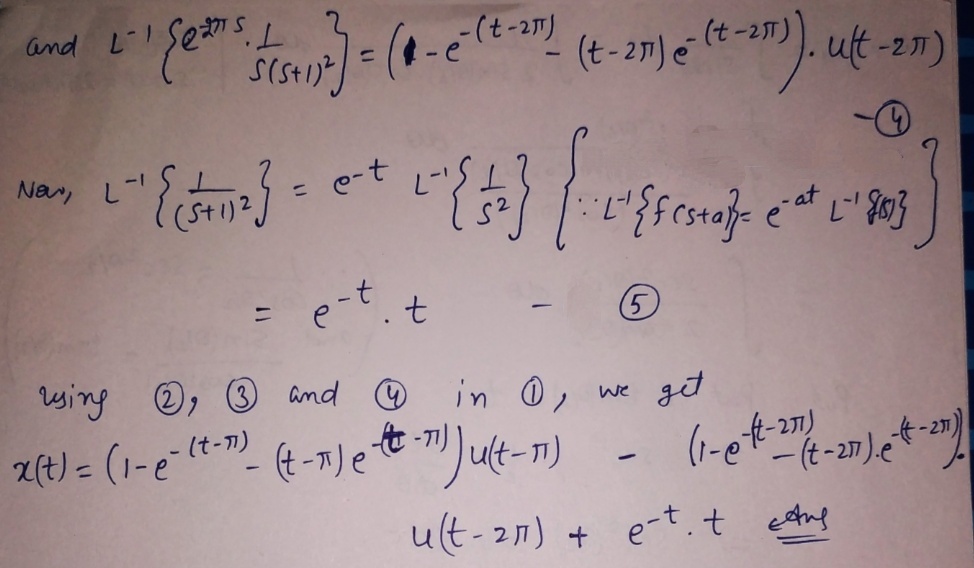##### Add Answer of: 3. Use Laplace transforms to solve the following initial value problems. Write the solution (t) f...
Similar Homework Help Questions
• ### Use Laplace transforms to solve the following initial value problems. Where possible, describe the solution behavior...

Use Laplace transforms to solve the following initial value problems. Where possible, describe the solution behavior in terms of oscillation and decay. y′′ +4y = δ(t−1), y(0) = 3, y′(0) = 0.

• ### Use Laplace Transforms to solve the initial value problems...? Will rate lifesaver.

Use Laplace Transforms to solve the initial value problem.x^(11)= x double primex(1)=x prime10.) x^(11) +3x^(1) +2x = t ; x(0)=0 and x^(1)(0)=2I know the answer => x(t)=(1/4)(2t -3 +12e^(-t) -9e^(-2t)), butI do not know how to get that answer. Please show step by stepprocess. I will rate lifesaver.You might need the Laplace transforms table to answer. And youdefinitely use integrals.

• ### Please show all work and write clear. Use Laplace transforms to solve the following initial value...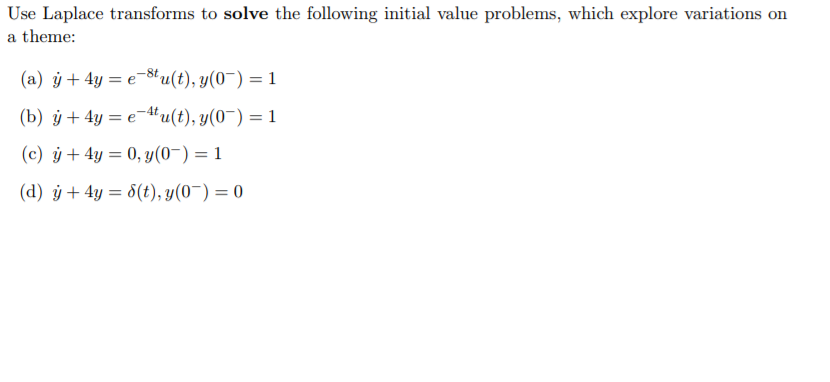Please show all work and write clear. Use Laplace transforms to solve the following initial value problems, which explore variations on a theme: (c) +4y0,01

• ### 15) 5. Use Laplace transforms to solve the initial value problem y" + y = g(t),...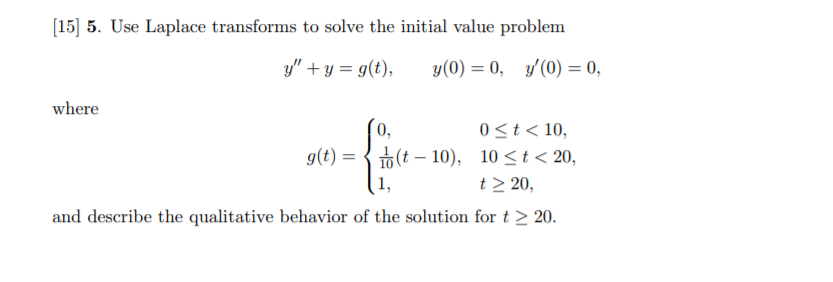15) 5. Use Laplace transforms to solve the initial value problem y" + y = g(t), y'(0) = 0, y(0) = 0, where 0 St< 10, 10 t 20, 0, g(t) = (t-10), 1, t < 20, and describe the qualitative behavior of the solution fort 20

• ### use laplace transforms to solve the initial value problem

Use Laplace Transforms to solve the initial value problemy'' + y = 3 sin(2t)-3 sin(2t)u(t-2pi), y(0)=1 , y'(0)= -2First find the laplace transform Y(s) of y(t)Y(s)=Now find y(t)y(t)=

• ### 7.6.27 Solve the given initial value problem using the method of Laplace transforms. z"' + 6z'...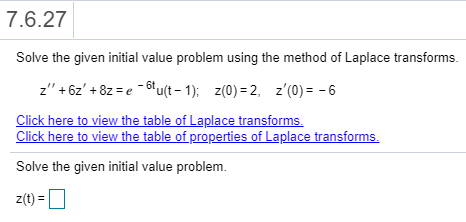7.6.27 Solve the given initial value problem using the method of Laplace transforms. z"' + 6z' + 8z = e-bu(t-1); Z(0) = 2, z'(0) = -6 Click here to view the table of Laplace transforms. Click here to view the table of properties of Laplace transforms Solve the given initial value problem. z(t)=

• ### Laplace transforms to solve Initial Value Problems1. Use Laplace transforms to solve the initial value problem -a) b) c)

• ### Laplace Transforms and Initial Value Problems

Use Laplace transforms to solve the initialvalue problems.1. x'' + 8x' + 15x = 0; x(0) = 2, x'(0) = -32. x'' + 4x = cost; x(0) = 0 = x'(0)3. x'' + 9x = 1; x(0) = 0 = x'(0)

• ### Laplace transforms

Use Laplace transforms to solve the initial value problemsx''+2x+4y=0y''+x+2y=0x(0)=y(0)=0x'(0)=y'(0)=-1

• ### 5) Use the method of Laplace transforms to the solve the following boundary value problem IC: u(x...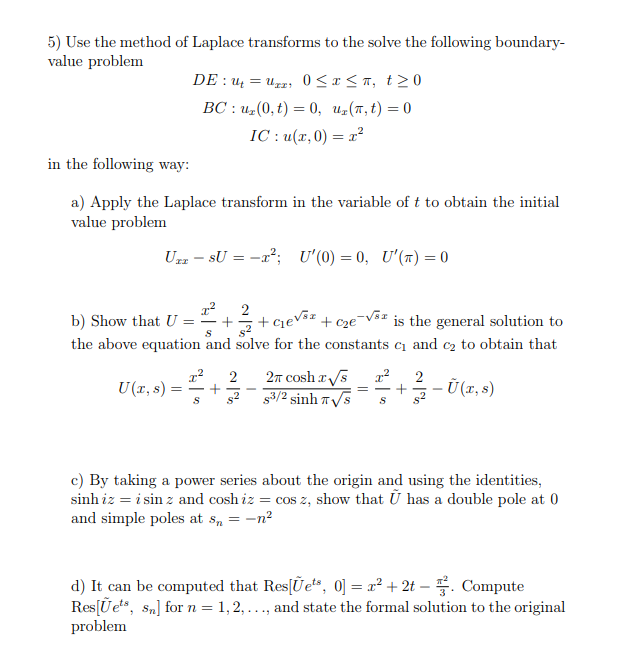5) Use the method of Laplace transforms to the solve the following boundary value problem IC: u(x, 0) 2 in the following way: a) Apply the Laplace transform in the variable of t to obtain the initial value problem b) Show that U =-+ cie'sz +cge-Vsz s the general solution to the above equation and solve for the constants c and c2 to obtain that c) By taking a power series about the origin and using the identities, sinh iz-...

Need Online Homework Help?